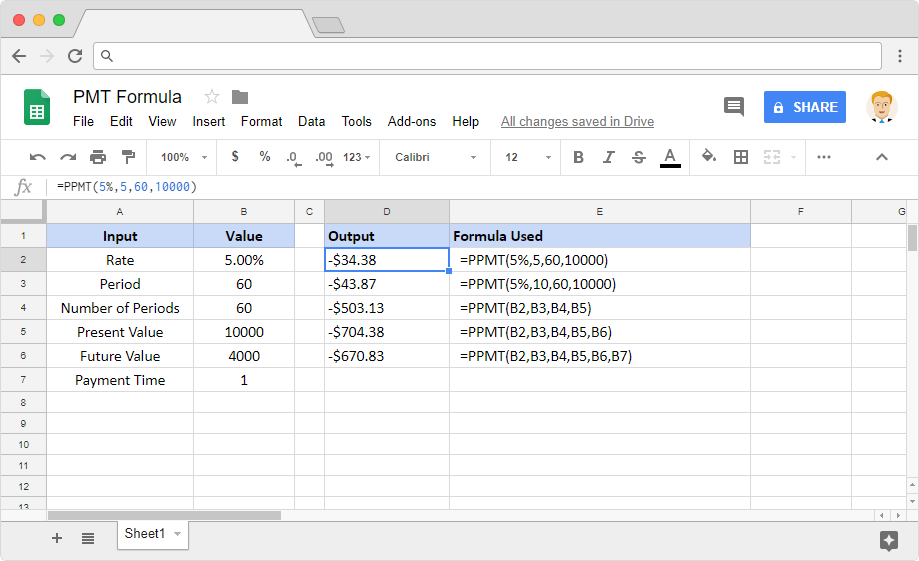# How to use the PPMT formula in Google SheetsAlmost all of us at some point in our lives may opt for loans, against a prevailing interest rate. And we’d be interested to know the principal we pay over the course of the repayment schedule. How do we calculate that? The PPMT formula in Google Sheets can help us here – it stands for Principal Payment. The investment in question is based on a constant interest rate and also a constant-amount periodic payment schedule.

### Syntax

PPMT(rate, period, number_of_periods, present_value, [future_value, end_or_beginning])

• rate – is the rate of interest.
• period – is the amortization period that we want to calculate the Principal payment for. The least value can be 1 and the maximum value can be equal to the number_of_periods.
• number_of_periods – is the number of periods that the buyer wishes to make payment for.
• present_value – is the current value of the annuity.
• future_value – [OPTIONAL] – is the amount of the future value that remains after we make the final payment.
• end_or_beginning – [OPTIONAL – 0 by default] – a 0 indicates that we are making the payments at the end of each period. And a value of 1 specifies that we are making payments at the beginning of each payment period.

### Usage: PPMT formula in Google Sheets

Now that we know what the PPMT formula is used for along with its syntax, let’s dive head-first into the practical application. With the help of a few examples, that is. Please have a look at the following image.One thing we know about the amortization schedule is that we start paying more interest in the initial months than the later months. And the principal repayment is the opposite – we pay more at the end of the overall period than we do in the initial months.

We can clearly identify this pattern in the first three formula examples. The 5th-period principal payment is less than the 10th-period payment, which in turn is much less than the 60th-period principal payment.

#### Note

We’d encourage you to try and understand the multiple variations of these formulas we’ve illustrated as examples. Analyze how each and every parameter value is affecting the output values. While we are at this, the PMT formula might interest you as well. It calculates the overall payment (principal + interest) amount for an amortization period.